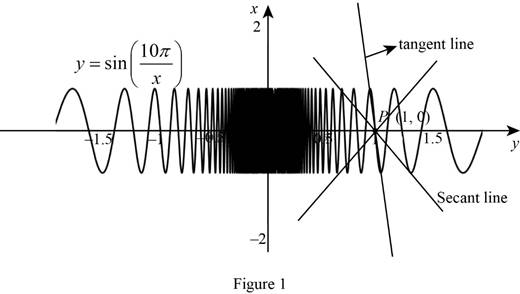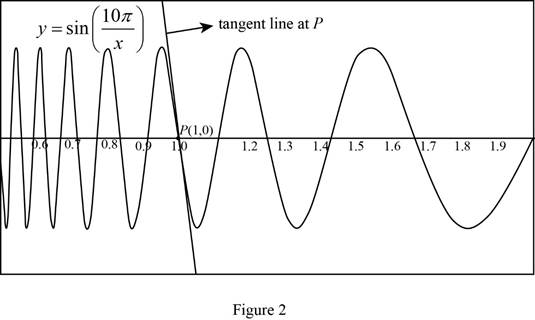# The slope of the secant line PQ for the given values of x.### Single Variable Calculus: Concepts...

4th Edition
James Stewart
Publisher: Cengage Learning
ISBN: 9781337687805### Single Variable Calculus: Concepts...

4th Edition
James Stewart
Publisher: Cengage Learning
ISBN: 9781337687805

#### Solutions

Chapter 2.1, Problem 9E

(a)

To determine

## To find: The slope of the secant line PQ for the given values of x.

Expert Solution

The slope of the secant line PQ for the following values of x is given below:

 x Slope mPQ 2 0 1.5 1.7321 1.4 −1.0847 1.3 −2.7433 1.2 −4.3301 1.1 −2.8173 0.5 0 0.6 −2.1651 0.7 −2.6061 0.8 −5 0.9 3.4202

### Explanation of Solution

Given:

The equation of the curve is y=sin(10πx).

The point P(1, 0) lies on the curve y.

The Q is the point (x,sin(10πx)).

Calculation:

The slope of the secant lines between the points, P(1, 0) and Q(x,sin(10πx)) is

mPQ=sin(10πx)0x1 (1)

Obtain the slope of the secant line PQ for the value of x=2.

Substitute 2 for x in sin(10πx),

sin(10πx)=sin(10π2)=sin(5π)=0

Substitute Q(x,sin(10πx))=(2,0) in equation (1),

mPQ=sin(10πx)0x1=0021=01=0

Thus, the slope of the secant line PQ for the value of x=2 is 0.

Obtain the slope of the secant line PQ for the value of x=1.5.

Substitute 1.5 for x in sin(10πx),

sin(10πx)=sin(10π1.5)=sin(203π)=0.866025

Substitute Q(x,sin(10πx))=(1.5,0.866025) in equation (1),

mPQ=sin(10πx)0x1=0.86602501.51=0.8660250.51.7321

Thus, the slope of the secant line PQ for the value of x=1.5 is 1.7321.

Obtain the slope of the secant line PQ for the value of x=1.4.

Substitute 1.4 for x in sin(10πx),

sin(10πx)=sin(10π1.4)=0.43388

Substitute Q(x,sin(10πx))=(1.4,0.43388) in equation (1),

mPQ=sin(10πx)0x1=0.4338801.41=0.433880.41.0847

Thus, the slope of the secant line PQ for the value of x=1.4 is 1.0847.

Obtain the slope of the secant line PQ for the value of x=1.3.

Substitute 1.3 for x in sin(10πx).

sin(10πx)=sin(10π1.3)=0.82298

Substitute Q(x,sin(10πx))=(1.3,0.82298) in equation (1).

mPQ=sin(10πx)0x1=0.8229801.31=0.822980.32.7433

Thus, the slope of the secant line PQ for the value of x=1.3 is 2.7433.

Obtain the slope of the secant line PQ for the value of x=1.2.

Substitute 1.2 for x in sin(10πx),

sin(10πx)=sin(10π1.2)=0.86602

Substitute Q(x,sin(10πx))=(1.2,0.86602) in equation (1),

mPQ=sin(10πx)0x1=0.8660201.21=0.866020.24.3301

Thus, the slope of the secant line PQ for the value of x=1.2 is 4.3301.

Obtain the slope of the secant line PQ for the value of x=1.1.

Substitute 1.1 for x in sin(10πx),

sin(10πx)=sin(10π1.1)=0.28173

Substitute Q(x,sin(10πx))=(1.1,0.28173) in equation (1),

mPQ=sin(10πx)0x1=0.2817301.11=0.281730.12.8173

Thus, the slope of the secant line PQ for the value of x=1.1 is 2.8173.

Obtain the slope of the secant line PQ for the value of x=0.5.

Substitute 0.5 for x in sin(10πx),

sin(10πx)=sin(10π0.5)=sin(20π)=0

Substitute Q(x,sin(10πx))=(0.5,0) in equation (1),

mPQ=sin(10πx)0x1=000.51=00.50

Thus, the slope of the secant line PQ for the value of x=0.5 is 0.

Obtain the slope of the secant line PQ for the value of x=0.6.

Substitute 0.5 for x in sin(10πx),

sin(10πx)=sin(10π0.6)=0.86603

Substitute Q(x,sin(10πx))=(0.6,0.86603) in equation (1),

mPQ=sin(10πx)0x1=0.8660300.61=0.866030.42.1651

Thus, the slope of the secant line PQ for the value of x=0.6 is 2.1651.

Obtain the slope of the secant line PQ for the value of x=0.7.

Substitute 0.7 for x in sin(10πx).

sin(10πx)=sin(10π0.7)=0.78183

Substitute Q(x,sin(10πx))=(0.7,0.78183) in equation (1).

mPQ=sin(10πx)0x1=0.7818300.71=0.781830.32.6061

Thus, the slope of the secant line PQ  for the value of x=0.7 is 2.6061.

Obtain the slope of the secant line PQ  for the value of x=0.8.

Substitute 0.8 for x in sin(10πx),

sin(10πx)=sin(10π0.8)=1

Substitute Q(x,sin(10πx))=(0.8,1) in equation (1).,

mPQ=sin(10πx)0x1=100.81=10.25

Thus, the slope of the secant line PQ for the value of x=0.8 is 5.

Obtain the slope of the secant line PQ for the value of x=0.9.

Substitute 0.9 for x in sin(10πx),

sin(10πx)=sin(10π0.9)=0.34202

Substitute Q(x,sin(10πx))=(0.9,0.34202) in equation (1),

mPQ=sin(10πx)0x1=0.3420200.91=0.342020.13.4202

Thus, the slope of the secant line PQ for the value of x=0.9 is 3.4202.

Conclusion:

The slope does not appear to be approaching a limit. Suppose x approaches 1, then the slope mPQ do not approach  any specific value.

(b)

To determine

Expert Solution

### Explanation of Solution

The graph of the curve y=sin(10πx) is shown below in Figure 1.From Figure 1, it is observed that there seems to be the frequent oscillations of the graph. Moreover, the tangent line is so steep at the point P(1, 0). Thus, the slopes of the secant lines are not closer to the slope of the tangent line at P. Therefore, it is necessary to consider the values of x much closer to 1 for better accurate estimates of the slope.

(c)

To determine

### To estimate: The slope of the tangent line to the curve at P(1, 0).

Expert Solution

The estimated value of the slope of the tangent line to the curve at P (1, 0) is −31.4.

### Explanation of Solution

The graph of the curve y=sin(10πx) between the points 0.5 and 2 is shown below in Figure 2.The secant line is close to the tangent line at P(1, 0) when x=0.999 and x=1.001.

The value of the slope of the tangent line to the curve at P(1,0) is close to the average value of the slope of the secant lines closest to P.

Obtain the slope of the secant line PQ for the value of x=1.001.

Substitute 1.001 for x in sin(10πx).

sin(10πx)=sin(10π1.001)=0.031379

Substitute Q(x,sin(10πx))=(1.001,0.031379) in equation (1).

mPQ=sin(10πx)0x1=0.031379401.0011=0.03137940.00131.3794

Thus, the slope of the secant line PQ for the value of x=1.001 is 31.3794.

Obtain the slope of the secant line PQ for the value of x=0.999.

Substitute 0.999 for x in sin(10πx).

sin(10πx)=sin(10π0.999)=0.03144219

Substitute Q(x,sin(10πx))=(0.999,0.03144219) in equation (1).

mPQ=sin(10πx)0x1=0.0314421900.9991=0.031442190.00131.4422

Thus, the slope of the secant line PQ for the value of x=0.999 is 31.4422.

The slope of the secant line PQ for the value of x=1.001 is 31.3794 and the slope of the secant line PQ for the value of x=0.999 is 31.4422.

Take the average of two slopes of the secant lines,

m31.3794+(31.4422)262.8216231.410831.4

Thus, the estimated value of the slope of the tangent line to the curve at P (1, 0) is −31.4.

### Have a homework question?

Subscribe to bartleby learn! Ask subject matter experts 30 homework questions each month. Plus, you’ll have access to millions of step-by-step textbook answers!